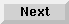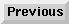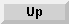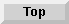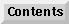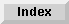14 Integrating C Functions (DLL)

## Generating a DLL

This section presumes you have experience with Eloquence and C, and that you have previously worked with HP-UX tools.

First write the C functions you want to call from your Eloquence application.

Then start the program /usr/eloquence/dllcc. This program analyzes the C functions and determines the function names and arguments which have to be used in the CALL DLL statement. This information is stored in a second C source file.

Now compile all C source files using the ANSI-C option and link them with the library libeloq.a. LOAD DLL starts the DLL process and initializes the shared memory.

The DLL program is started as a separate process from within Eloquence to prevent mutual interference.

The DLL process now waits for a signal from Eloquence. CALL DLL copies the arguments into shared memory and signals the DLL process that the arguments have been passed. Now control is passed to your C function. The DLL process converts the passed arguments into the format specified in the C functions. If the return value of the C function is non-zero, this value is used to force an Eloquence error. If no error has occured the DLL function transfers the arguments passed as "passed by reference" back to the shared memory segment and signals the Eloquence process that the DLL function has finished.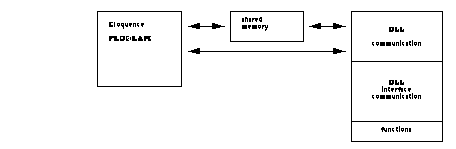NOTE: ANSI-C functionality is not supported by the C-compiler provided as part of the standard HP-UX operating sytem. It is supported by the C-compiler sold as an optional, separate product. See the example in the directory /usr/eloquence/example of how to compile a DLL without the optional ANSI-C compiler. The example DLL described in this manual is generated using the ANSI-C compiler.

### DLLCC

dllcc [options] {- | files }

Options:

-help
Gives usage
-l
Output source listing
-o file
Output file name, default is stdout
Specifying input file '-' will force reading from stdin

Examples:

```   dllcc -o sampleif.c sample1.c sample2.c
cat test.c | dllcc - >testif.c
```
Each source file containing functions to interface with Eloquence must include the file dllif.h.

Each user function you want to call from Eloquence must be of type EqDLL.

The function arguments must be of the following type:

EqVoid
this function has no arguments
EqInt
argument is of type Integer (long)
EqReal
argument is of type Real (double)
EqChar
argument is of type String, terminated by a NULL-character (char *)
EqString
argument is of type String (EqSring *)
...
the function has a variable number of arguments
The DLLCC program supports the following syntax:

C comments (/*...*/) are recognized and ignored.

The C preprocessor statements

```#if 0 ... #endif
```
can be used to deactivate whole segments, but they must not be nested.

The function syntax must follow the ANSI-C convention:

```     EqDLL functionname ( EqVoid )
EqDLL functionname ( Argument [, Argument] )
EqDLL functionname ( Argument [, Argument [,...]] , ...)
```
Argument types:

```     EqInt ArgumentName
```
This is an Integer argument which cannot be modified ("pass by value"). Valid argument types are INTEGER, DINTEGER, SHORT, REAL

```     EqInt *ArgumentName
```
This is an Integer argument which can be modified or an Integer array ("pass by reference"). Valid argument types are INTEGER, DINTEGER

```     EqInt ArgumentName size
```
This is an Integer array ("pass by reference"). If size is specified, this array must at least have size elements. Valid argument types are INTEGER, DINTEGER.

```     EqReal ArgumentName
```
This is a Real argument which cannot be modified ("pass by value"). Valid argument types are INTEGER, DINTEGER, SHORT, REAL.

```     EqReal *ArgumentName
```
This is a Real argument which can be modified, or a Real array ("pass by reference"). Valid argument types are SHORT, REAL.

```      EqReal ArgumentName size
```
This is a Real array ("pass by reference"). If size is specified, this array must at least have size elements. Valid argument types are SHORT, REAL

```      EqChar *ArgumentName
```
This is a pointer to a zero-terminated character array ("pass by value"). Valid argument type is STRING.

```      EqString *ArgumentName
```
This is a String argument which can be modified or a String array ("pass by reference"). Valid argument type is STRING.

```      EqString ArgumentName size
```
This is a String array ("pass by reference"). If size is specified, this array must have at least size elements. Valid argument type is STRING

```      ...
```
This is a variable argument ("pass by reference"). Type depends on the Eloquence data type passed. A maximum of 20 arguments can be passed.

#### Example

This example shows a C program with the definition of two functions that could be called from an Eloquence application, and the output file generated by the dllcc program, the makefile and the corresponding Eloquence program.

Example:

```/* num.c */

#include "dllif.h"

EqDLL int_add( EqInt int1, EqInt int2, EqInt *result )
{
*result = int1 + int2;
return(0);
}

EqDLL int_div( EqInt int1, EqInt int2, EqInt *result )
{
if(int2 == 0)
return(31);           /* division by zero */
*result = int1 / int2;
return(0);
}
```

The call

```   dllcc -o num.if.c num.c
```
causes dllcc to generate the file num.if.c with the following content:

Example:

```/*
num.if.c
THIS FILE IS GENERATED AUTOMATICALLY BY DLLCC
DON'T CHANGE MANUALLY
*/

#include <dllif.h>

/*
int_add( long int1, long int2, long *result )
int_div( long int1, long int2, long *result )
*/

struct EqFuncList EqFuncList[] = {
{ "int_div",   3, 3 },
{ 0L }
};

struct EqArgList EqArgList[] = {
{ "int1",      EqArg_IntByValue        , 0 },
{ "int2",      EqArg_IntByValue        , 0 },
{ "result",    EqArg_IntByReference    , 0 },
{ "int1",      EqArg_IntByValue        , 0 },
{ "int2",      EqArg_IntByValue        , 0 },
{ "result",    EqArg_IntByReference    , 0 },
};

int EqCall(fn, av)
int fn; void *av[];
{
switch(fn) {
case 0:
return int_add(*(EqInt *)(av), *(EqInt *)(av), (EqInt *)(av));
case 1:
return int_div(*(EqInt *)(av), *(EqInt *)(av), (EqInt *)(av));
default:
return -1;
}
}
```

This is the sample 'makefile' to build the above example.

Example:

```# compiler flags
CFLAGS = -Aa +OV

Num: num.o num.if.o
\$(CC) -o \$@ \$(CFLAGS) num.if.o num.o -leloq -lmalloc

num.if.o: num.c
/usr/eloquence/dllcc -o num.if.c num.c
\$(CC) \$(CFLAGS) -c -o \$@ num.if.c
rm -f num.if.c
```

The corresponding Eloquence application is NUM.PROG.

Example:

```1000 ! RE-STORE "NUM,EXAMPLE"
1010 !
1020     INTEGER Res
1030 !
1040     ON ERROR GOSUB Error
1050 !
1070 !
1090     PRINT Res
1100     CALL DLL Num("int_div",5,2,Res)
1110     PRINT Res
1120     CALL DLL Num("int_div",1,0,Res)
1130     PRINT Res                     ! Value unchanged due to error
1140 !
1150     DEL DLL Num
1160     END
1170 !
1180 Error:!
1190     PRINT ERRM\$
1200     PRINT ERRMSG\$(ERRN)
1210     RETURN
```

### Programming Guidelines

#### DLL interface level

All source files using DLL interface level functions must include the file dllif.h. It defines the data types and the function prototypes.

```  #include dllif.h
```

#### DLL interface level functions

```
struct EqFuncInfo *EqFuncInfo( void )
```
This function returns information about the current function.

Type EqFuncInfo is defined in dllif.h.

```   struct EqFuncInfo {
char *name;
int arg_cnt;
};
```
name contains a pointer to the function name.

arg_cnt contains the number of arguments.

This data must not be modified.

```   struct EqArgInfo *EqArgInfo( void *arg )
```
This function returns information about a function argument. If the argument is a pointer, it can be used to find out further information on this argument. Alternatively, the order (beginning at 0) can be passed.

If the argument is invalid, NULL is returned.

Type EqArgInfo is defined in dllif.h.

```    struct EqArgInfo {
enum EqArgType type;
char *name;
int elcnt;
};

```
type specifies the argument type.

name contains a pointer to the name of the argument. For a variable argument this is "...".

elcnt contains the number of elements. Unless the argument is an array, this is 1.

This data must not be modified.

int EqStrlen ( EqString *s);

Returns current string length of Eloquence string

int EqMaxStrlen( EqString *s);

Returns maximum string length of Eloquence string

int EqStrcat( EqString *s1, EqString *s2);

Appends a copy of string s2 to the end of string s1. Returns zero on success or 18 on string overflow.

int EqStrcpy( EqString *s1, EqString *s2);

Copies string s2 to string s1. Returns zero on success or 18 on string overflow.

int EqStrcmp( EqString *s1, EqString *s2);

Compares its arguments and returns an integer less than, equal to, or greater than zero, depending on whether string s1 is lexicographically less than, equal to, or greater than string s2.

EqString *EqSubstr( EqString *s, int start, int len);

Extracts substring of Eloquence string.

start is the position of the first character starting at 1

len is the number of characters to be extracted.

Returns new Eloquence string descriptor, or NULL pointer if arguments are invalid.

NOTE: The return value points to a static area which will be overwritten on next call. The string value is not moved; the descriptor will point into original string.

int EqStr2str( EqString *eq, void *s);

Converts Eloquence string into zero terminated string. Returns string length without trailing zero.

NOTE: Target string must provide enough space to hold string value and terminating \0 character.

int str2EqStr( void *s, EqString *eq);

Converts zero terminated string into Eloquence string. Returns zero on success, or 18 on string overflow.

EqString *EqMkstr( int maxl);

Allocate new string using malloc(). Returns NULL on memory allocation failure.

NOTE: The return value points to a static area and will be overwritten on next call.

#### Setup/cleanup hooks

The functions dll_setup() and dll_cleanup() are optional. If specified by the programmer, they are called when the DLL process starts, or before it terminates.

```   void dll_setup( void )
void dll_cleanup( void )
```
The DLL process can be terminated in dll_setup() or dll_cleanup() using exit().

To terminate the process while inside a function called by Eloquence, the process should send itself a SIGTERM signal (e.g. raise(SIGTERM); ). This is necessary to clean up properly.

#### Signals

The following signals are used internally and MUST NOT be modified:

SIGINT
ignored
SIGTERM
is rerouted to the DLL termination handler
SIGUSR2
is rerouted to the DLL manager
The signal handling is internally realized using the sigvector functionality.

#### Environment

When a DLL is called, all files with the exception of stderr are closed.

Stderr is linked to the terminal but not integrated into the Eloquence screen buffer.

If you reroute stderr when starting Eloquence, for example for the purpose of external tracing, all output onto stderr will also be rerouted into this file.

#### Debugging

If you set the global variable dll_debug, you can print out the call, the arguments and return value of the function called into stderr.

DLL debug levels

0 = no debugging

1 = function call and return value

2 = function call, argument values, return value

3 = internal

#### xdb

To debug a DLL process with xdb, follow the procedure below:

Make sure that the file .xdbrc is present in your HOME directory, and that it contains the following:

```   z 17 rs
```
All modules of the DLL process must be compiled and linked using the debug option (-g).

You will need 2 terminals or 2 windows. No further user can have loaded the DLL.

Now start the Eloquence program on terminal 1. After the LOAD DLL statement has been executed, you can look up the process number of the DLL process using the 'ps' command.

Now start xdb on terminal 2 using the following options:

```   xdb -P {DLL process number} {DLL filename}
```
Insert a breakpoint in the routine(s) you want to test and continue the DLL process with xdb 'c' command.

Now continue the Eloquence program execution on the first terminal. After the CALL DLL statement you are in debug mode.

#### DLL communication level

NOTE: Do not use the DLL communication level unless absolutely necessary. Using the DLL communication level is more trouble, more prone to errors and generally not so flexible. Using the DLL communication level is not possible in conjunction with the DLL interface level.

3 functions are available:

```   int dll_main( void )
```
dll_main() is called in a CALL DLL. The return code is interpreted as an Eloquence runtime error number.

```   void dll_init( void )
void dll_exit( void )
```
These functions are called when the DLL process is started or terminated.

#### DLL communication level utilities

When using DLL communication level, there are 3 functions available:

```   int u_get_argc( void )
```
Returns the number of Eloquence arguments.

```   t_DLL_arg u_get_arg(int idx)
```
Returns the description of an Eloquence argument.

```   u_unref_arg(int idx)
```
Flags an argument passed as "pass by reference" as not modified (for TRACE).

Example:

```/*
This is a sample DLL communication level routine
It will force eloquence runtime error with the error
number given as argument.
*/

#include <dll.h>

int dll_main( void )
{
int argc;
t_DLL_arg arg;

/* get argument count */
argc = u_get_argc();
if(argc != 1)
return(9);

/* check for integer argument */
arg = u_get_arg(0);
if(arg.type != DLL_INTEGER)
return(8);
if(arg.elcnt != 1)
return(8);

/* return error number */
return(*(int *)arg.ptr);
}
```

Eloquence Language Manual - 19 DEC 2002